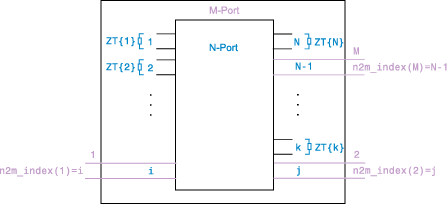# snp2smp

Convert and reorder single-ended N-port S-parameters to single-ended M-port S-parameters

## Syntax

``s_params_mp = snp2smp(s_params_np)``
``s_params_mp = snp2smp(s_params_np,Z0,n2m_index,ZT)``
``s_params_mp = snp2smp(s_obj,n2m_index,ZT)``

## Description

example

````s_params_mp = snp2smp(s_params_np)` convert and reorder the single-ended N-port S-parameters, `s_params_np`, into the single-ended M-port S-parameters, `s_params_mp`. M must be less than or equal to N.```

example

````s_params_mp = snp2smp(s_params_np,Z0,n2m_index,ZT)` convert and reorder the S-parameter data using the optional arguments `Z0`, `n2m_index`, and `ZT` that control the conversion.The following figure illustrates how to use the optional input arguments to specify the ports for the output data and the termination of the remaining ports.```
````s_params_mp = snp2smp(s_obj,n2m_index,ZT)` convert and reorder a S-parameters object, `s_obj`, into the single-ended M-port S-parameters, `s_params_mp`. M must be less than or equal to N.```

## Examples

collapse all

Convert 3-port S-parameters to 3-port S-parameters with port indices swapped from `[1 2 3]` to `[2 3 1]` .

`ckt = read(rfckt.passive,'default.s3p');`

Default.s3p represents a real counterclockwise circulator.

```s3p = ckt.NetworkData.Data; Z0 = ckt.NetworkData.Z0; s3p_new = snp2smp(s3p,Z0,[2 3 1]); s3p_new = s3p_new(1:5)```
```s3p_new = 1×5 complex 0.1431 - 0.7986i 0.0898 + 0.3177i -0.0318 + 0.4208i -0.0701 + 0.4278i 0.0503 - 0.8080i ```

Convert 3-port S-parameters to 2-port S-parameters by terminating port 3 with an impedance of `Z0`.

```ckt = read(rfckt.passive,'default.s3p'); s3p = ckt.NetworkData.Data; Z0 = ckt.NetworkData.Z0; s2p = snp2smp(s3p,Z0); s2p_new = s2p(1:5)```
```s2p_new = 1×5 complex -0.0073 - 0.8086i 0.0869 + 0.3238i -0.0318 + 0.4208i 0.1431 - 0.7986i -0.0330 - 0.8060i ```

Convert 16-port S-parameters to 4-port S-parameters by using ports `1`, `16`, `2`, and `15` as the first, second, third, and fourth ports. Terminate the remaining 12 ports with an impedance of `Z0`.

```S = sparameters('default.s16p'); s16p = S.Parameters; Z0 = S.Impedance; s4p = snp2smp(s16p,Z0,[1 16 2 15],Z0); s4p = s4p(:,:,1)```
```s4p = 4×4 complex 0.0857 - 0.1168i -0.5372 - 0.6804i 0.0966 - 0.0706i 0.0067 + 0.0053i -0.5366 - 0.6860i 0.0803 - 0.1234i 0.0059 + 0.0048i 0.0977 - 0.0703i 0.0957 - 0.0700i 0.0067 + 0.0048i 0.0818 - 0.1104i -0.5362 - 0.6838i 0.0055 + 0.0051i 0.0972 - 0.0703i -0.5376 - 0.6840i 0.0761 - 0.1180i ```

Convert 16-port S-parameters to 4-port S-parameters by using ports `1`, `16`, `2`, and `15` as the first, second, third, and fourth ports terminate port `4` with an impedance of `100` ohms and terminate the remaining `11` ports with an impedance of `50` ohms.

```S = sparameters('default.s16p'); s16p = S.Parameters; Z0 = S.Impedance; ZT(1:16) = {50}; ZT{4} = 100; s4p = snp2smp(s16p,Z0,[1 16 2 15],ZT); s4p(:,:,1)```
```ans = 4×4 complex 0.0857 - 0.1168i -0.5372 - 0.6804i 0.0966 - 0.0706i 0.0067 + 0.0053i -0.5366 - 0.6860i 0.0803 - 0.1234i 0.0059 + 0.0048i 0.0977 - 0.0703i 0.0957 - 0.0700i 0.0067 + 0.0048i 0.0818 - 0.1104i -0.5362 - 0.6838i 0.0055 + 0.0051i 0.0972 - 0.0703i -0.5376 - 0.6840i 0.0761 - 0.1180i ```

## Input Arguments

collapse all

S-parameters, specified as a N-by-N-by-K array , where K representing number of frequency points of a N-port S-parameters.

S-parameter object, specified as N-port scalar handle objects, which include numeric arrays of S-parameters.

Reference impedance of N-port S-Parameters, specified as positive real scalar in ohms.

`n2m_index` is a vector of length M specifying how the ports of the N-port S-parameters map to the ports of the M-port S-parameters. `n2m_index(i)` is the index of the port from `s_params_np` that the function converts to the `i`th port of `s_params_mp`. For example, the setting `[1, 2]` means that M is `2`, and the first two ports of the N-port S-parameters become the ports of the M-port parameters. The function terminates any additional ports with the impedances specified by `ZT`.

Termination Impedance of the ports,`ZT`, specified as a scalar, vector, or cell array. If M is less than N, `snp2smp` terminates the NM ports not listed in `n2m_index` using the values in `ZT`. If `ZT` is a scalar, the function terminates all NM ports not listed in `n2m_index` by the same impedance `ZT`. If `ZT` is a vector of length K, `ZT[i]` is the impedance that terminates all NM ports of the `i`th frequency point not listed in `n2m_index`. If `ZT` is a cell array of length N, `ZT{j}` is the impedance that terminates the `j`th port of the N-port S-parameters. The function ignores impedances related to the ports listed in `n2m_index`. Each `ZT{j}` can be a scalar or a vector of length K.

## Output Arguments

collapse all

Single-ended M-port S-parameters, returned as one of the following:

• If you provide `s_params_np` as an input, `s_params_mp` is returned as a M-by-M-by-K array representing K M-port S-parameters. where M representing number of frequency points of a single-ended M-port S-Parameters.

• If you provide `s_obj` as an input, `s_params_mp` is returned as a S-parameter object with following properties:

• `NumPorts` — Number of ports, specified as an integer. The function calculates this value automatically when you create the object.

• `Frequencies` — S-parameter frequencies, specified as a K-by-1 vector of positive real numbers sorted from smallest to largest. The function sets this property from the `filename` or `freq` input arguments.

• `Parameters` — S-parameter data, specified as an N-by-N-by-K array of complex numbers. The function sets this property from the `filename` or `data` input arguments.

• `Impedance` — Reference impedance in ohms, specified as a positive real scalar. The function sets this property from the `filename` or `Z0` input arguments. If no reference impedance is provided, the function uses a default value of `50`.

Complex Number Support: Yes

## Version History

Introduced in R2007b